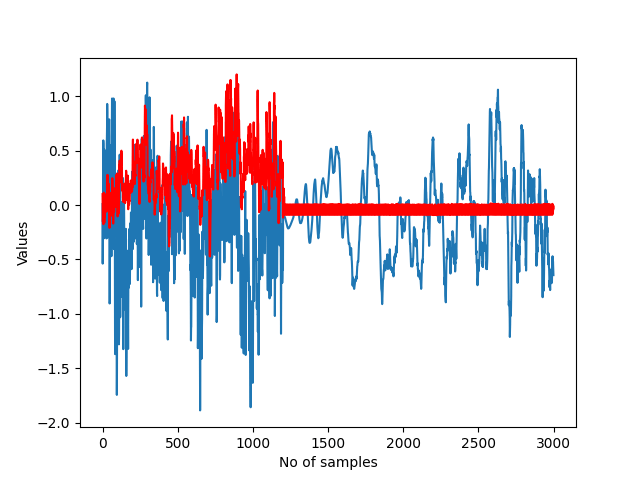# #StackBounty: #time-series #autoencoder #anomaly-detection #overfitting #matplotlib Autenocoder and anomaly detection task

### Bounty: 50

I’m trying to create an autoencoder for the anomaly detection task, but I’m noticing that even if it performs very well on the training set, it starts to stop recreating half of the test set. I tried with more than 10 models, (LSTM, ConvAE, ConvLSTM) and all of them fails to reconstruct the time series in the same point.

These are the performances on the training set. The blue part is the original time series and the red one is the time series reconstructed by the AE.These are the performance on the training set. I don’t understand why all the models stop performing from that point. Could that means that there are anomalies in that part?EDIT: I’m updating the question with some details about my dataset and my code: I have a dataset with 30 devices, and for each one I have about 9000 values. The dataset is structured as well:

``````device1   device2   device3   ....   device30
0.20      0.35      0.12              0.56
1.20      2.10      5.75              0.16
3.20      9.21      1.94              5.12
5.20      4.32      0.42              9.56
....      ....      ....              ....
7.20      6.21      0.20              -9.56
``````

Since I’m following this guide, I started creating a sequence method to prepare my data for the Conv1D layer:

``````TIME_STEPS = 10
# Generated training sequences for use in the model.
def create_sequences(values, time_steps=TIME_STEPS):
output = []
for i in range(len(values) - time_steps):
output.append(values[i: (i + time_steps)])
return np.stack(output)
``````

This is where I create the sequences and normalize the dataset:

``````# split the train/val/test set
n_features = dataset_sequences.shape
X_train = dataset_sequences[0:3000, :]
X_val = dataset_sequences[3000:6000, :]
X_test = dataset_sequences[6000:9000, :]

# normalize the data
train_mean = X_train.mean()
train_std = X_train.std()
X_train = (X_train - train_mean) / train_std
X_val = (X_val - train_mean) / train_std
X_test = (X_test - train_mean) / train_std
``````

Then, I feed my X_train with shape (3000, 10, 30) to my Conv1D autoencoder:

``````model = tf.keras.Sequential(
[
tf.keras.layers.Input(shape=(X_train.shape, X_train.shape)),
tf.keras.layers.Conv1D(
tf.keras.layers.Dropout(rate=0.2),
tf.keras.layers.Conv1D(
tf.keras.layers.MaxPooling1D(pool_size=2),
tf.keras.layers.Conv1DTranspose(